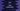# Java program to add, subtract, multiply and divide using switch case## Java program to add, subtract, multiply and divide using switch case:

In this post, we will learn how to write a simple calculator program in Java using switch cases. The program can add, subtract, multiply and divide two user input numbers. It will use switch case to find the calculation.

With this program, you will learn how to take user inputs, how to use switch cases and how to do basic mathematical calculations in Java.

### Syntax of switch statement:

The statement of switch case is defined as below:

``````switch(expression){
case value1:
// body
break;
case value2:
// body
break;
...
...
default:
// body
}``````
• We can use switch cases variables in int, long, enum, short, byte, strings, Byte, Short, Int and Long. It is similar to if else-if statements.
• We can have any number of case in a switch statement.
• The value in the case statements should be a literal or constant.
• If any value of a case is equal to the result of the expression, the statements of the body of the case are executed.
• The break statement is optional. It will exit from the switch block and move the control to the next line.
• If no break statement is defined, it will move to the next switch statement.
• The default statement is optional. The body of default is executed if it fails for all case values.

### Method 1: Java program to add, subtract, multiply and divide by using switch blocks:

Let’s take a look at the below program:

``````import java.util.Scanner;

class Example {
public static void main(String[] args) {
char c;
int firstNumber, secondNumber, output = 0;

Scanner scanner = new Scanner(System.in);

System.out.println("Enter + for addition, - for subtraction, * for multiplication and / for division: ");
c = scanner.next().charAt(0);

System.out.println("Enter the first number: ");
firstNumber = scanner.nextInt();

System.out.println("Enter the second number: ");
secondNumber = scanner.nextInt();

switch (c) {
case '+':
output = firstNumber + secondNumber;
break;
case '-':
output = firstNumber - secondNumber;
break;
case '*':
output = firstNumber * secondNumber;
break;
case '/':
output = firstNumber / secondNumber;
break;
default:
System.out.println("Please enter a valid symbol!!");
return;
}

System.out.printf("%d %s %d = %d", firstNumber, c, secondNumber, output);
}
}``````

Here,

• The character variable c is used to store the calculation symbol. Valid characters are +, -, * and / for addition, subtraction, multiplication and division respectively. It asks the user to enter the symbol, reads it and store it in the variable c.
• The switch statement works based on the value of c.
• It has four cases and one default block. If the value of c is valid, it calculates the result and stores it in the variable output. If it is not valid, it prints a message to enter a valid symbol and returns.
• The last printf statement prints the result.

It will give output as below:

``````Enter + for addition, - for subtraction, * for multiplication and / for division:
+
Enter the first number:
23
Enter the second number:
33
23 + 33 = 56

Enter + for addition, - for subtraction, * for multiplication and / for division:
-
Enter the first number:
22
Enter the second number:
10
22 - 10 = 12

Enter + for addition, - for subtraction, * for multiplication and / for division:
*
Enter the first number:
8
Enter the second number:
12
8 * 12 = 96

Enter + for addition, - for subtraction, * for multiplication and / for division:
/
Enter the first number:
111
Enter the second number:
11
111 / 11 = 10

Enter + for addition, - for subtraction, * for multiplication and / for division:
&
Enter the first number:
12
Enter the second number:
33
Please enter a valid symbol!!``````

### Method 2: Java program to add, subtract, multiply and divide by using switch blocks and a separate function:

Let’s use a separate method to calculate the result. The program will call this method to get the result. It will take the calculation symbol, first number and the second number as its parameters and return the calculated value.

``````import java.util.Scanner;

class Example {
private static int calculate(char c, int firstNumber, int secondNumber) {
switch (c) {
case '+':
return firstNumber + secondNumber;
case '-':
return firstNumber - secondNumber;
case '*':
return firstNumber * secondNumber;
case '/':
return firstNumber / secondNumber;
default:
return -1;
}
}

public static void main(String[] args) {
char c;
int firstNumber, secondNumber, output;

Scanner scanner = new Scanner(System.in);

System.out.println("Enter + for addition, - for subtraction, * for multiplication and / for division: ");
c = scanner.next().charAt(0);

System.out.println("Enter the first number: ");
firstNumber = scanner.nextInt();

System.out.println("Enter the second number: ");
secondNumber = scanner.nextInt();

output = calculate(c, firstNumber, secondNumber);

if (output == -1) {
System.out.println("Please enter a valid symbol!!");
} else {
System.out.printf("%d %s %d = %d", firstNumber, c, secondNumber, output);
}
}
}``````

Here,

• We created a new function calculate that calculates the result.
• This method takes the calculation symbol, first number and the second number as its parameters and returns the result value.
• We are not using any break statement in the case blocks. It returns the calculated value. For the default statement, it returns -1.
• The returned value is assigned to the output variable and based on its value, it prints either a error message or the calculated result.